#### Higher dimensional manifolds

The construction techniques used for the 2D manifolds generalize nicely to higher dimensions. Of course,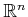, is an-dimensional manifold. An-dimensional torus,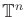, can be made by taking a Cartesian product ofcopies of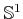. Note that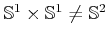. Therefore, the notationis used for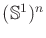. Different kinds of-dimensional cylinders can be made by forming a Cartesian product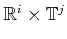for positive integersand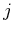such that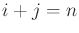. Higher dimensional spheres are defined as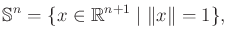(4.7)

in which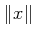denotes the Euclidean norm of, andis a positive integer. Many interesting spaces can be made by identifying faces of the cube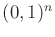(or even faces of a polyhedron or polytope), especially if different kinds of twists are allowed. An-dimensional projective space can be defined in this way, for example. Lens spaces are a family of manifolds that can be constructed by identification of polyhedral faces .

Due to its coming importance in motion planning, more details are given on projective spaces. The standard definition of an-dimensional real projective space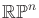is the set of all lines in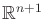that pass through the origin. Each line is considered as a point in. Using the definition of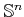in (4.7), note that each of these lines inintersects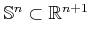in exactly two places. These intersection points are called antipodal, which means that they are as far from each other as possible on. The pair is also unique for each line. If we identify all pairs of antipodal points of, a homeomorphism can be defined between each line through the origin ofand each antipodal pair on the sphere. This means that the resulting manifold,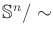, is homeomorphic to.

Another way to interpret the identification is thatis just the upper half of, but with every equatorial point identified with its antipodal point. Thus, if you try to walk into the southern hemisphere, you will find yourself on the other side of the world walking north. It is helpful to visualize the special case of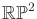and the upper half of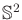. Imagine warping the picture offrom Figure 4.5 from a square into a circular disc, with opposite points identified. The result still represents. The center of the disc can now be lifted out of the plane to form the upper half of.

Steven M LaValle 2020-08-14